Detail Result Created Edited

# Local Direction Force Sum

## Function

• Local Direction Force Sum provides internal forces on a selected line or plane in beam, plate and solid elements. The resultant forces and the acting point are calculated on the basis of all the nodal forces included in the selected plane.
• Once a detail analysis is carried out for a specific part of the structure, this function conveniently calculates the member forces for structural design.

## Call

From main menu, select [Results] tab > [Detail] group > [Beam/Element] >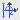[Local Direction Force Sum]

## Input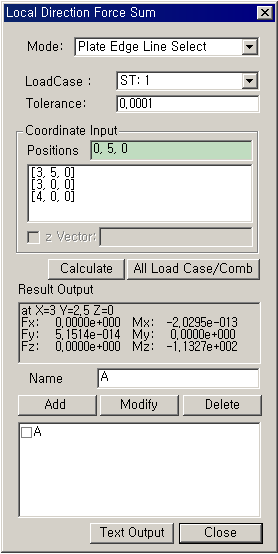### Mode

Assign the method of selecting the subject plane that includes the nodes where internal forces are to be combined

Solid Face Polygon Select
Define an arbitrary polygon to specify the faces of solid elements contained within that polygon.

Plate Edge Line Select
Define an arbitrary line to specify the edges of plate elements that coincide with that line.

Plate Edge Polygon Select
Define an arbitrary polygon to specify the edges of plate elements that are in contact with that polygon.

### Tolerance

Tolerance defining a line or polygon

### Coordinate Input

Positions : Enter the GCS coordinates of the points necessary to assign a line or polygon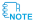Enter the coordinates of the points, or click the entry field and the desired points to automatically enter the coordinates. To select Plate Edge Line Select, enter the coordinates of the two points defining a line and additionally enter the coordinates of the 3rd point that defines the direction as to which side of the elements are to be considered.

z Vector : Enter a vector to define the z-axis of the local coordinate system to which Result Output is referenced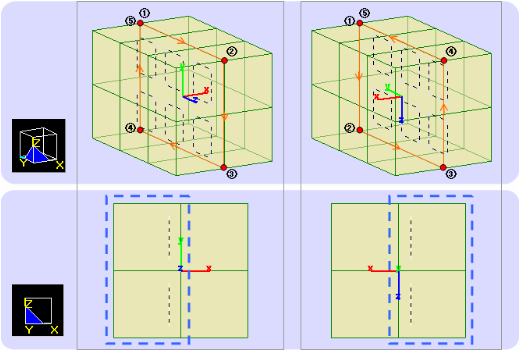Solid Face Polygon

(The sectional forces are calculated using the nodal forces of the elements marked with dashed lines.)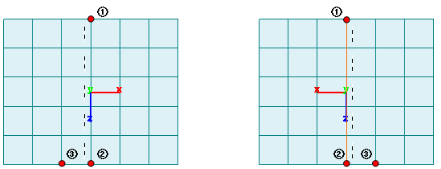Plate Edge Line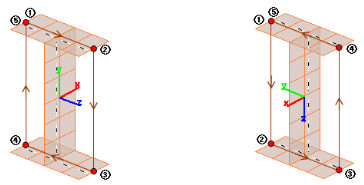Plate Edge PolygonThe sum of nodal internal forces for each element is calculated at the center of the selected plane section with respect to the local coordinate system. When defining a polygon, the local axes are determined by the entry sequence of the coordinates of the points (right hand rule). If the z-vector is not defined, the direction from the 1st to 2nd point of the polygon becomes the local z-axis by default. Click [Apply] in the Local Direction Force Sum dialog box to review the local coordinate axes displayed at the centroid.

### Result Output

Produce the position of the centroid of the defined section and the sum of internal forces for each direction.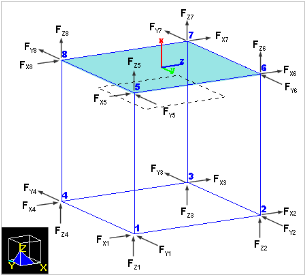The sectional forces are calculated using the element forces at the user-defined region's nodal points. The left figure provides an example of calculating sectional forces for a single solid element.

The forces in each direction are equal to the sum of the element forces in that direction at the nodal points.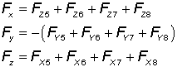The calculation method for the moments in each direction is as follows, where dy5 represents the distance in the y-direction from the centroid to nodal point 5.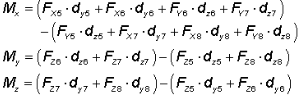### Name

Enter a name and click the Add button to save the specified area information and sectional forces.

After selecting the saved sectional forces information with the "Check on" option, click the Text Output button to save the sectional forces results.

0